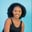Related Tags

python3
pandas

# How to use the Series.transform() function in pandasMaria Elijah

## Overview

The pandas Series.transform() function applies a function on itself and produces a Series of the transformed element with the same axis shape as itself.

### Syntax

Series.transform(func, axis=0)


### Parameters:

• func: This represents the function or list of functions used to transform the series elements.
• axis: This represents the parameter needed for DataFrame compatibility. It can be 0 or index.

### Code example

The following code will demonstrate how to use the Series.transform() function in pandas:

import pandas as pd

# create Series
my_series = pd.Series([17, 10, 28, 15, 23, 7, 9, 36, 14])

# apply lambda function to series element
# using product()
print(my_series.transform(lambda x: x **2 - 100))
print(len(my_series))

### Code explanation

In the code above:

• Line 1: We import the pandas library.

• Lines 4: We create Series called my_series of length 9.

• Line 8: We pass a lambda function as an argument to the transform(), which multiplies each element in the Series by the power of 2 and subtracts 100 from each.

RELATED TAGS

python3
pandas

CONTRIBUTORMaria Elijah
RELATED COURSES

View all Courses

Keep Exploring

Learn in-demand tech skills in half the time